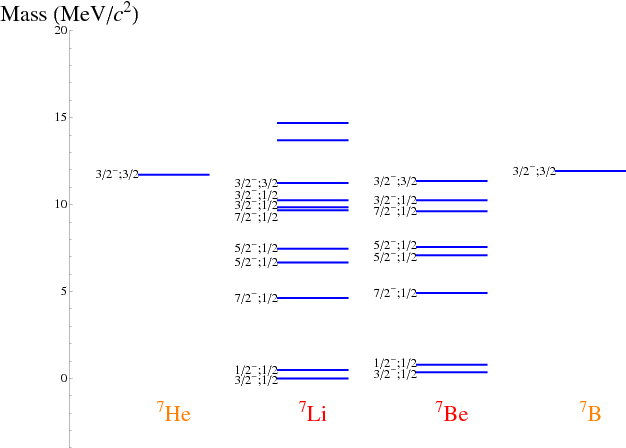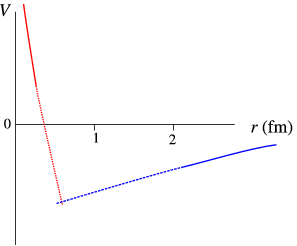$$\require{cancel}$$

# 3.6: Properties of Nuclear States

•• Contributed by Niels Walet
• Professor (Physics) at University of Manchester

Nuclei are quantum systems, and as such must be described by a quantum Hamiltonian. Fortunately nuclear energies are much smaller than masses, so that a description in terms of non-relativistic quantum mechanics is possible. Such a description is not totally trivial since we have to deal with quantum systems containing many particles. Rather then solving such complicated systems, we often resort to models.

## Quantum numbers

As in any quantum system there are many quantum states in each nucleus. These are labelled by their quantum numbers, which, as will be shown later, originate in symmetries of the underlying Hamiltonian, or rather the underlying physics.

### Angular Momentum

One of the key invariances of the laws of physics is rotational invariance, i.e., physics is independent of the direction you are looking at. This leads to the introduction of a vector angular momentum operator,

$\hat{\vec{L}} = \hat{\vec{r}} \times \hat{\vec{p}},$

which generates rotations. As we shall see later quantum states are not necessarily invariant under the rotation, but transform in a well-defined way. The three operators $$\hat{L}_x$$, $$\hat{L}_y$$ and $$\hat{L}_z$$ satisfy a rather intriguing structure,

$[\hat{L}_x,\hat{L}_y] \equiv \hat{L}_x \hat{L}_y -\hat{L}_y \hat{L}_x = i\hbar \hat{L}_z,$

and the same for cyclic permutation of indices ($$xyz\rightarrow yzx$$ or $$zxy$$). This shows that we cannot determine all three components simultaneously in a quantum state. One normally only calculates the length of the angular momentum vector, and its projection on the $$z$$ axis,

\begin{aligned} \hat{\vec{L}}^2 \phi_{LM} &= \hbar^2 L(L+1) \phi_{LM},\nonumber\\ \hat L_z \phi_{LM} &= \hbar L_z \phi_{LM}.\end{aligned}

It can be shown that $$L$$ is a non-negative integer, and $$M$$ is an integer satisfying $$|M|<L$$, i.e., the projection is always smaller than or equal to the length, a rather simple statement in classical mechanics.

The standard, albeit slightly simplified, picture of this process is that of a fixed length angular momentum precessing about the $$z$$ axis, keeping the projection fixed, as shown in Figure $$\PageIndex{1}$$.Figure $$\PageIndex{1}$$: A pictorial representation of the “quantum precession” of an angular momentum of fixed length $$L$$ and projection $$M$$.

The energy of a quantum state is independent of the $$M$$ quantum number, since the physics is independent of the orientation of $$L$$ in space (unless we apply a magnetic field that breaks this symmetry). We just find multiplets of $$2L+1$$ states with the same energy and value of $$L$$, differing only in $$M$$.

Unfortunately the story does not end here. Like electrons, protons and neutron have a spin, i.e., we can use a magnetic field to separate nucleons with spin up from those with spin down. Spins are like orbital angular momenta in many aspects, we can write three operators $$\hat{\vec{S}}$$ that satisfy the same relation as the $$\hat{L}$$’s, but we find that

\begin{aligned} \hat{\vec{S}}^2 \phi_{S,S_z}= \hbar^2 \frac{3}{4} \phi_{S,S_z},\end{aligned}

i.e., the length of the spin is $$1/2$$, with projections $$\pm1/2$$.

Spins will be shown to be coupled to orbital angular momentum to total angular momentum $$J$$,

$\hat{\vec{J}}=\hat{\vec{L}}+\hat{\vec{S}},$

and we shall specify the quantum state by $$L$$, $$S$$, $$J$$ and $$J_z$$. This can be explained pictorially as in Figure $$\PageIndex{2}$$.Figure $$\PageIndex{2}$$: A pictorial representation of the vector addition of spin and orbital angular momentum.

There we show how, for fixed length $$J$$ the spin and orbital angular momentum precess about the vector $$\vec{J}$$, which in its turn precesses about the $$z$$-axis. It is easy to see that if $$\vec{L}$$ and $$\vec{S}$$ are fully aligned we have $$J=L+S$$, and if they are anti-aligned $$J=|L-S|$$. A deeper quantum analysis shows that this is the way the quantum number work. If the angular momentum quantum numbers of the states being coupled are $$L$$ and $$S$$, the length of the resultant vector $$J$$ can be

$J=|L-S|, |L-S|+2,\ldots,L+S.$

We have now discussed the angular momentum quantum number for a single particle. For a nucleus which in principle is made up from many particles, we have to add all these angular momenta together until we get something called the total angular momentum. Since the total angular momentum of a single particle is half-integral (why?), the total angular momentum of a nucleus is integer for even $$A$$, and half-integer for odd $$A$$.

### Parity

Another symmetry of the wavefunction is parity. If we change $$\vec{r} \rightarrow \vec{-r}$$, i.e., mirror space, the laws of physics are invariant. Since we can do this operation twice and get back where we started from, any eigenvalue of this operation must be $$\pm1$$, usually denoted as $$\Pi=\pm$$. It can be shown that for a particle with orbital angular momentum $$L$$, $$\Pi=(-1)^L$$. The parity of many particles is just the product of the individual parities.

### isotopic spin (Isobaric spin, isospin)

The most complicated symmetry in nuclear physics is isospin. In contrast to the symmetries above this is not exact, but only approximate. The first clue of this symmetry come from the proton and neutron masses, $$m_n= \text{MeV}/c^2$$ and $$m_p= \text{MeV}/c^2$$, and their very similar behaviour in nuclei. Remember that the dominant binding terms only depended on the number of nucleons, not on what type of nucleons we are dealing with.

All of this leads to the assumption of another abstract quantity, called isospin, which describes a new symmetry of nature. We assume that both neutrons and protons are manifestation of one single particle, the nucleon, with isospin down or up, respectively. We shall have to see whether this makes sense by looking in more detail at the nuclear physics. We propose the identification

$Q = (I_z+1/2)e,$

where $$I_z$$ is the $$z$$ projection of the vectorial quantity called isospin.

Apart from the neutron-proton mass difference, isospin symmetry in nuclei is definitely broken by the Coulomb force, which acts on protons but not on neutrons. We shall argue that the nuclear force, that couples to the “nucleon charge” rather than electric charge, respects this symmetry. What we shall do is look at a few nuclei where we can study both a nucleus and its mirror image under the exchange of protons and neutrons. One example are the nuclei $$^7$$He and $$^7$$B (2 protons and 5 neutrons, $$I_z=-3/2$$ vs. 5 protons and 2 neutrons, $$I_z=3/2$$) and $$^7$$Li and $$^7$$B (3 protons and 4 neutrons, $$I_z=-1/2$$ vs. 4 protons and 3 neutrons, $$I_z=1/2$$), as sketched in Figure $$\PageIndex{3}$$.Figure $$\PageIndex{3}$$: The spectrum of the nuclei with $$A=7$$. The label of each state is $$J$$, parity, isospin. The zeroes of energy were determined by the relative nuclear masses.

We note there the great similarity between the pairs of mirror nuclei. Of even more importance is the fact that the $$3/2^-;3/2$$ level occurs at the same energy in all four nuclei, suggestion that we can define these states as an “isospin multiplet”, the same state just differing by $$I_z$$.

deuteron

Let us think of the deuteron (initially) as a state with $$L=0$$, $$J=1$$, $$S=1$$, usually denoted as $$^3S_1$$ ($$S$$ means $$L=0$$, the 3 denotes $$S=1$$, i.e., three possible spin orientations, and the subscript $$1$$ the value of $$J$$). Let us model the nuclear force as a three dimensional square well with radius $$R$$. The Schrödinger equation for the spherically symmetric $$S$$ state is (work in radial coordinates) $-\frac{\hbar^2}{2\mu} \frac{1}{r^2} \left(\frac{d}{d r}r^2 \frac{d}{dr} R(r) \right) +V(r)R(r)=E R(r).$ Here $$V(r)$$ is the potential, and $$\mu$$ is the reduced mass, $\mu=\frac{m_n m_p}{m_n + m_p},$ which arises from working in the relative coordinate only. It is easier to work with $$u(r)=rR(r)$$, which satisfies the condition

$-\frac{\hbar^2}{2\mu} \frac{d^2}{d r^2}u(r) +V(r)u(r)=E u(r),$

as well as $$u(0)=0$$. The equation in the interior

$-\frac{\hbar^2}{2\mu} \frac{d^2}{d r^2}u(r) V_0u(r)=E u(r),\qquad u(0)=0$

has as solution

$u = A\sin \kappa r,\qquad \kappa=\sqrt{\frac{2\mu}{\hbar^2}(V_0+E)}.$

Outside the well we find the standard damped exponential,

$u = B\exp (k r),\qquad k=\sqrt{\frac{2\mu}{\hbar^2}(-E)}.$

Matching derivatives at the boundary we find

$-\cot \kappa R = \frac{k}{\kappa} = \sqrt{\frac{-E}{V_0+E}}.$

We shall now make the assumption that $$|E|\ll V_0$$, which will prove true. Then we find

$\kappa \approx \sqrt{\frac{2\mu}{\hbar^2}V_0},\qquad \cot\kappa R \approx 0.$

Since it is known from experiment that the deuteron has only one bound state at energy $$-2.224573\pm0.000002 \text{ MeV}$$, we see that $$\kappa R \approx \pi/2$$! Substituting $$\kappa$$ we see that

$V_0 R^2 = \frac{\pi^2 \hbar^2}{8\mu}.$

If we take $$V_0=30\text{ MeV}$$, we find $$R=1.83\text{ fm}$$.

We can orient the spins of neutron and protons in a magnetic field, i.e., we find that there is an energy

$E_{magn} = \mu_N \mu {\vec{S}}\cdot {\vec{B}}.$

(The units for this expression is the so-called nuclear magneton, $$\mu_N=\frac{e\hbar}{2 m_p}$$.) Experimentally we know that

$\mu_n = -1.91315 \pm 0.00007 \mu_N\qquad \mu_p = 2.79271\pm0.00002 \mu_N$

If we compare the measured value for the deuteron, $$\mu_d = 0.857411\pm 0.000019 \mu_N$$, with the sum of protons and neutrons (spins aligned), we see that $$\mu_p +\mu_n= 0.857956\pm 0.00007 \mu_N$$. The close agreement suggest that the spin assignment is largely OK; the small difference means that our answer cannot be the whole story: we need other components in the wavefunction.

We know that an $$S$$ state is spherically symmetric and cannot have a quadrupole moment, i.e., it does not have a preferred axis of orientation in an electric field. It is known that the deuteron has a positive quadrupole moment of $$0.29 e^2\text{ fm}^2$$, corresponding to an elongation of the charge distribution along the spin axis.

From this we conclude that the deuteron wavefunction carries a small (7%) component of the $$^3D_1$$ state ($$D$$: $$L=2$$). We shall discuss later on what this means for the nuclear force.

## Scattering of Nucleons

We shall concentrate on scattering in an $$L=0$$ state only, further formalism just gets too complicated. For definiteness I shall just look at the scattering in the $$^3S_1$$ channel, and the $$^1S_0$$ one. (These are also called the triplet and singlet channels.)

## Nuclear Forces

Having learnt this much about nuclei, what can we say about the nuclear force, the attraction that holds nuclei together? First of all, from Rutherford’s old experiments on $$\alpha$$ particle scattering from nuclei, one can learn that the range of these forces is a few fm.

From the fact that nuclei saturate, and are bound, we would then naively build up a picture of a potential that is strongly repulsive at short distances, and shows some mild attraction at a range of 1-2 fm, somewhat like sketched in Figure $$\PageIndex{4}$$.Figure $$\PageIndex{4}$$: Possible form for the internucleon potential, repulsive at short distances, and attractive at large distances.

Here we assume, that just as the Coulomb force can be derived from a potential that only depends on the size of $$r$$,

$V(r)= \frac{q_1 q_2}{4\pi\epsilon_0 r},$

the nuclear force depends only on $$r$$ as well. This is the simplest way to construct a rotationally invariant energy. For particles with spin other possibilities arise as well (e.g., $$\vec{S} \cdot \vec{r}$$) so how can we see what the nuclear force is really like?

Since we have taken the force to connect pairs of particles, we can just study the interaction of two nucleons, by looking both at the bound states (there is only one), and at scattering, where we study how a nucleon gets deflected when it scatters of another nucleon. Let us first look at the deuteron, the bound state of a proton and a neutron. The quantum numbers of its ground state are $$J^\pi=1^+$$, $$I=0$$. A little bit of additional analysis shows that this is a state with $$S=1$$, and $$L=0$$ or $$2$$. Naively one would expect a lowest state $$S=0$$, $$L=0$$ (which must have $$I=$$ for symmetry reasons not discussed here). So what can we read of about the nuclear force from this result?

We conclude the following:

1. The nuclear force in the $$S$$ waves is attractive.
2. Nuclear binding is caused by the tensor force.
3. The nuclear force is isospin symmetric (i.e., it is independent of the direction of isospin).